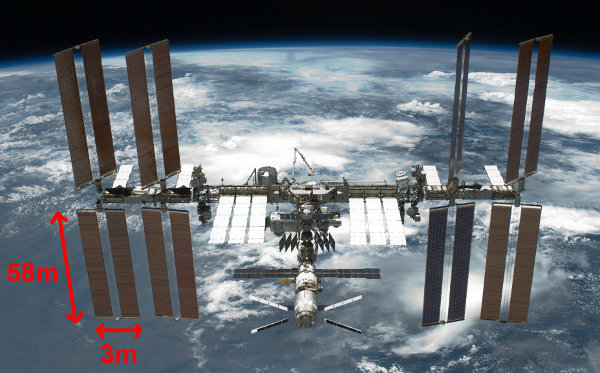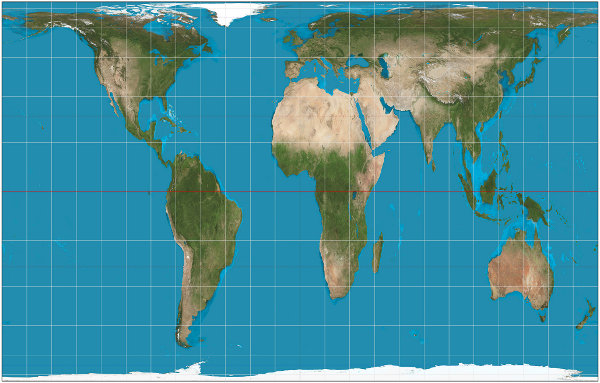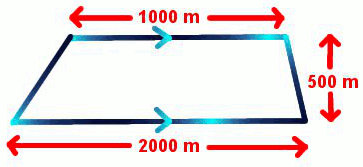Area is calculated when we want to find the flat surface to:

• collect sunshine for solar panels
• paint a wall
• tile a bathroom
• carpet a floor
• spread grass seeds to grow a lawn
• fertilize a garden

A Quadrilateral is a four-sided shape such as a rectangle, a square, a parallelogram and a trapezoid.

The area rules for each of these is based on the Area of Rectangle rule and are:

Area of Rectangle = L × W

Area of Square = S × S

Area of Parallelogram = L × H

 Area of Trapezoid = (A + B) × H 2

CONVERTING AREA UNITS

To change:

• square centimetres (cm2) to square millimetres (mm2), multiply by 10 and then multiply again by 10 (or multiply by 100).
• square metres (m2) to square millimetres (mm2), multiply by 1000 and by 1000 again (or multiply by 1 000 000).
• square metres (m2) to square centimetres (cm2), multiply by 100 and by 100 again (or multiply by 10 000).
• square kilometres (km2) to square metres (m2), multiply by 1000 and by 1000 again (or multiply by 1 000 000).

IF YOU SQUARE THE NAME, YOU SQUARE THE NUMBER.

## Example One - Area of RectangleThe International Space Station has been continuously occupied by astronauts performing experiments in astronomy, biology, chemistry and physics for over 12 years. It has four double-sided photo-voltaic arrays (solar panels) to make electricity. Each of the 8 panels measures 58 metres long by 3 metres wide. What is its total sunlight collection area?

Area of Rectangle
= L × W
= 58 × 3
= 174 square metres or 174 m2

Area of 8 Rectangular Panels
= 174 × 8
= 1392 square metres or 1392 m2

## Did You Know That...?The commonly used world map is misleading when displaying the actual area of countries. The Gall-Peters Map Projection shown above is more accurate.

## Example Two - Area of SquareWhat is the tiled area of a square bathroom floor with a side of 3000mm?
(Remember carpenters measure accurately in millimetres, but tiles are priced by the square metre.)

Area of Square
= S × S
= 3 × 3
= 9 m2

## Example Three - Area of Parallelogram

One thousand postage stamps in the shape of a parallelogram are printed. Each stamp has a length of 25 mm and a perpendicular height (at right angles to the length) of 12 mm. What is the area of glue (on the reverse side) to cover 1000 stamps?Area of Parallelogram
= L × H
= 25 × 12
= 300 mm2

Area of glue on 1000 stamps
= 300 × 1000
= 300 000 mm2 or 3000 cm2

## Example Four - Area of Trapezoid

A farm in the shape of a trapezoid and has parallel sides of 1000 metres and 2000 metres respectively. The perpendicular height is 500 metres. What is the area (in hectares) of this property? (Hint: 1 hectare = 10 000 square metres)Area of Trapezoid = (A + B) × H 2
 = (1000 + 2000) × 500 2

= 1500 × 500
= 750 000 m2
= 75 hectares

## Example Five - Painting a Wall

Calculate the quantity of paint required to paint a rectangular bedroom wall 4 metres by 2.4 metres. Two coats of paint are needed. One litre of paint covers 10m2.

Area (1 coat)
= L × W
= 4 × 2.4
= 9.6 m2

Area (2 coats)
= 9.6 × 2
= 19.2 m2

Number of litres
= 19.2 / 10
= 1.92
= 2 litres (Remember that you can't buy parts of litres of paint from the hardware store.)

## Questions

Find the areas of the following quadrilaterals. Be careful to convert the dimensions to the same units first.

Q1. Square with a side of 7 metres
Q2. Rectangle with a length of 10km and a width of 700 metres
Q3. Parallelogram with a length of 5 metres and a perpendicular height of 60cm
Q4. Trapezoid with parallel sides of 10cm and 6cm, and a perpendicular height of 5cm

A1. 49 m2
A2. 21.4 km2 or 7000000 m2
A3. 3 m2 or 30000 cm2
A4. 5 cm2

## Maths Fun - Trapezoidal Numbers

Trapezoidal Numbers (also called Polite Numbers) are the sum of consecutive positive integers. Examples of Trapezoidal Numbers are:

• 1 + 2 = 3
• 1 + 2 + 3 = 6
• 2 + 3 + 4 = 9
• 3 + 4 + 5 + 6 = 18
• 10 + 11 + 12 + 13 = 46

Why is the number 4 "impolite"? Why do you think these are called "Trapezoidal"? (Hint: Think of arranging blocks like steps.)